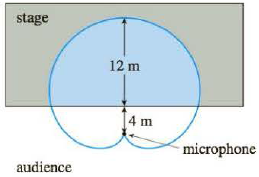Chapter 10.4, Problem 44E

Chapter
Section
Textbook Problem

When recording live performances, sound engineers often use a microphone with a cardioid pickup pattern because it suppresses noise from the audience. Suppose the microphone is placed 4 m from the front of the stage (as in the figure) and the boundary of the optimal pickup region is given by the cardioid r = 8 + 8 sin θ, where r is measured in meters and the microphone is at the pole. The musicians want to know the area they will have on stage within the optimal pickup range of the microphone. Answer their question.To determine

To Find: The area on the stage within the optimal pickup range of the microphone.

Explanation

Given:

The Boundary of the optimal pickup region is given by the cardioid.

r=8+8sinθ (1)

The microphone is placed at location of 4m from the front of the stage.

y=4 (2)

Calculation:

Substitute 0 for θ in the equation (1).

r=8+8sin(0)=8

Calculate the value of x.

x=rcosθ

Substitute 8 for r and 0 for θ .

x=8×cos(0×π180)=8

Calculate the value of y.

y=rsinθ

Substitute 8 for r and 0 for θ .

y=8×sin(0×π180)=0

Repeat the calculation of the values of x and y using the value of θ from 0 to 360 .

Tabulate the values of x and y in table (1).

 θ r=8+8sinθ x=rcosθ y=rsinθ 0.00 8.00 8.00 0.00 10.00 9.39 9.25 1.63 20.00 10.74 10.09 3.67 30.00 12.00 10.39 6.00 40.00 13.14 10.07 8.45 50.00 14.13 9.08 10.82 60.00 14.93 7.46 12.93 70.00 15.52 5.31 14.58 80.00 15.88 2.76 15.64 90.00 16.00 0.00 16.00 100.00 15.88 -2.76 15.64 110.00 15.52 -5.31 14.58 120.00 14.93 -7.46 12.93 130.00 14.13 -9.08 10.82 140.00 13.14 -10.07 8.45 150.00 12.00 -10.39 6.00 160.00 10.74 -10.09 3.67 170.00 9.39 -9.25 1.63 180.00 8.00 -8.00 0.00 190.00 6.61 -6.51 -1.15 200.00 5.26 -4.95 -1.80 210.00 4.00 -3.46 -2.00 220.00 2.86 -2.19 -1.84 230.00 1.87 -1.20 -1.43 240.00 1.07 -0.54 -0.93 250.00 0.48 -0.17 -0.45 260.00 0.12 -0.02 -0.12 270.00 0.00 0.00 0.00 280.00 0.12 0.02 -0.12 290.00 0.48 0.17 -0.45 300.00 1.07 0.54 -0.93 310.00 1.87 1.20 -1.43 320.00 2.86 2.19 -1.84 330.00 4.00 3.46 -2.00 340.00 5.26 4.95 -1.80 350.00 6.61 6.51 -1.15 360.00 8.00 8.00 0.00

Graph:

The graph is plotted for x and y using the table (1) as shown in figure (1).

Convert equation (2) into polar equation.

Substitute rsinθ for y in the equation (2).

rsinθ=4

r=4sinθ (3)

Equate the equation (1) and (3).

4sinθ=8+8sinθ4=sinθ(8+8sinθ)4=8sinθ+8sin2θ0=8sinθ+8sin2θ40=4(2sinθ+2sin2θ1)0=2sinθ+2sin2θ1

Solve the above equation using quadratic formula.

x=b±b24ac2a

Substitute sinθ for x , 2 for a, 2 for b and -1 for c in the above equation.

sinθ=2±(2)24(2)(1)2(2)=2±124=2±4×34=2±234

sinθ=1±32θ=sin1(1±32)

Here the value of θ=sin1(132) has no solution, hence ignore it.

θ=sin1(1+32)=sin1(0

Still sussing out bartleby?

Check out a sample textbook solution.

See a sample solution

The Solution to Your Study Problems

Bartleby provides explanations to thousands of textbook problems written by our experts, many with advanced degrees!

Get Started

Find more solutions based on key concepts